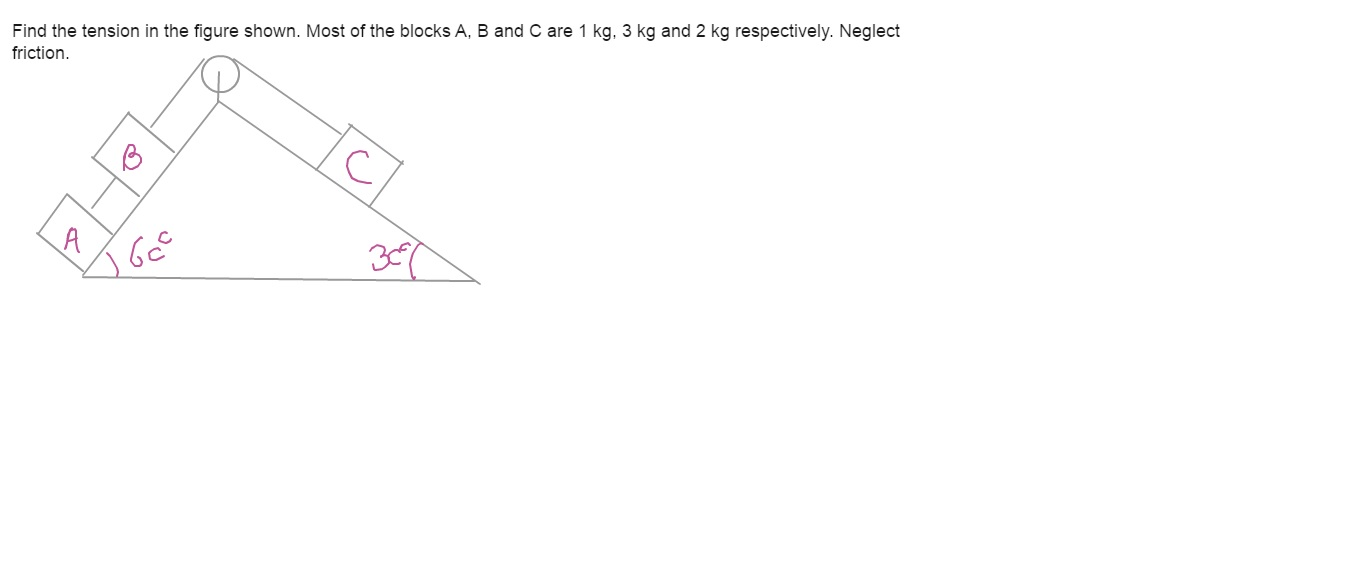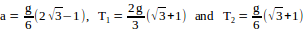# see attach7 years ago
p { margin-bottom: 0.25cm; line-height: 120%; }

Let

T1 = tension in the string connecting B and C

T2 = tension in the string connecting A and B

a = magnitude of acceleration of all the masses.

All of them will have same magnitude of acceleration because of spring length constraint.

From FBD of block A, mAgsin60o – T2 = mAa ie., gsin60o – T2 = a eq(1)

From FBD of block B, mBgsin60o + T2 – T1 = mBa ie., 3gsin60o + T2 – T1 = 3a eq(2)

From FBD of block C, T1 – mCgsin30o = mCa ie., T1 – 2gsin30o = 2a eq(3)

In writing the above equations we are assuming that acceleration of A and B is down the plane and C is up the plane.

Solving these equations, we get